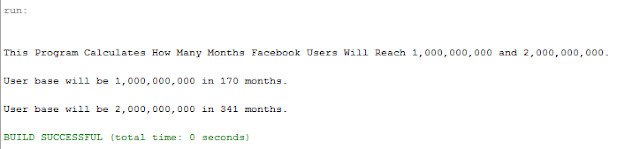# Facebook User Base Growth Calculator

The program below is the answer to Deitel's Java How to Program (9th Edition) Chapter 5 Exercise 5.32.

Question: According to CNNMoney.com, Facebook hit 500 million users in July of 2010 and its user base has been growing at a rate of 5% per month. Using the compound-growth technique you learned in Fig. 5.6 and assuming this growth rate continues, how many months will it take for Facebook to grow its user base to one billion users? How many months will it take for Facebook to grow its user base to two billion users (which, at the time of this writing, was the total number of people on the Internet)?

Solution: Using the technique (compound interest formula),
a = p + (1 + r)n
where a = amount
p = principal
r = rate
n = time (in years)

We are looking for how long the user base will grow to a certain number (time) so we make "n" the subject of the formula and convert "n" to months by multiplying by 12.
n = Math.log(amount/principal) / Math.log(1.0 + rate)
```/**
*
* @Author: Aghatise Osazuwa
* Website: www.cscprogrammingtutorials.com
*
* Exercise 5.32 - Facebook User Base Growth
*
*/

public class FBUserGrowth {
public static void main (String [] args) {
System.out.println("\n\nThis Program Calculates How Many Months Facebook Users Will Reach "
+ "1,000,000,000 and 2,000,000,000.");

double n;
double principal = 500000000.0; // initial amount before interest
double rate = 0.05;
double amount = 1000000000.0;
n = Math.log(amount/principal)/Math.log(1.0 + rate);
System.out.printf("\nUser base will be 1,000,000,000 in %d months.\n", Math.round(n*12));
amount = 2000000000.0;
n = Math.log(amount/principal)/Math.log(1.0 + rate);
System.out.printf("\nUser base will be 2,000,000,000 in %d months.\n\n", Math.round(n*12));
}
}
```Program Output

Click here to see other answers to Java How to Program.